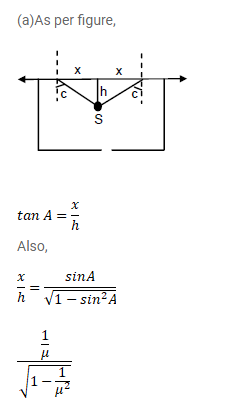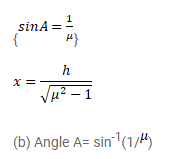# A point source is placed at a depth

Question:

A point source is placed at a depth $\mathrm{h}$ below the surface of water (refractive index= $\mu$ ). (a) Show that light escape through a circular area $n$ the water surface with its center directly above the point source. (b) Find the angle subtended by a radius of the area on the source.

Solution: